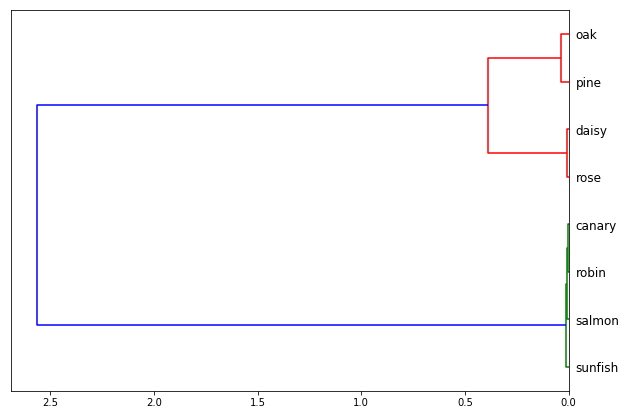# Building a classic feed-forward model in Keras/Tensorflow

This notebook describes the workflow for building a classic feed-forward neural network model using the Python libraries for Tensorflow and Keras (now bundled as part of the Tensorflow libraries). The notebook assumes you have installed tensorflow and keras in your Python installation and have some basic knowledge about programming in Python. I use Python in an Anaconda environment. Click here for a clear tutorial on installing Anaconda, Python, and Tensorflow.

For this tutorial we will build and train the Rumelhart model of semantic memory, which forms the basis for an influential theory of human knowledge representation in the mind and brain. The model was extensively analyzed in the 2004 book Semantic Cognition by me and Jay McClelland; a precis of the book was published here in Behavioral and Brain Sciences; a brief overview of the central ideas appears in this Nature Reviews Neuroscience article; and this more recent Nature Reviews article indicates how the model informs contemporary views on the cortical networks that support human conceptual knowledge. Finally, this tutorial briefly explains what the model does and describes how to build and test it using the Light Efficient Network Simulator.

# Import modules

First import the Python modules you will need:

numpy adds a variety of data-science tools, including the essential “array” data structure.

tensorflow is the library with methods and objects for building and fitting deep neural networks. It now includes Keras as part of the library.

In :
```import numpy as np
import tensorflow as tf
```

# Create and read training data

Before building the model it is useful to have the input and target patterns the network will process. Input and target patterns in Keras are stored in multi-dimensional arrays. An array is like a matrix, but where a matrix always has two dimensions (rows and columns), an array can have any arbitrary number of dimensions. If you are familiar with Excel workbooks, you can think of those as three-dimensional arrays. Each sheet has rows and columns but the workbook has a third dimension along which the individual worksheets are organized. You can specify any cell in the workbook with three pieces of information: row, column, and worksheet. Similarly, in a three-dimensional array, you can specify any cell in the array with three numbers; a 4D array requires 4 numbers, and so on. A matrix can be viewed as a 2D array, while a vector can be viewed as a 1D array. Python does not include arrays as a core data structure, but numpy does–so importing the numpy module is critical. It is standard to abbreviate this module as np.

For a classic feed-forward network, input patterns are stored in a 2D array (yes that is just a matrix but we will call it an array since that is the name of the Python data structure we will use). The first dimension of the array (the “rows”) indexes the different input items, while the 2nd dimension (columns) indexes the units in the input layer. For instance, the Rumelhart network has 8 “Item” input units and 32 different possible input patterns corresponding to all possible combinations of 8 items and 4 queries. So to store the input patterns for the Item layer, we need an array that has 32 rows and 8 columns.

The Item input patterns are coded as a one-hot vector. That is, each input pattern has one and only one Item input unit active, with all other units taking a value of 0. Since this is a very common way of coding information in a neural network, Keras and numpy provide tools for easily making one-hot vectors. Here is how I do it:

In :
```#Create array of item input values

i = np.arange(8)   #Array ranging from 0-7, indicating items 1-8. You need to start counting w 0!
i = np.concatenate((i,i,i,i))   #Repeat it 4 times, once for each context
I = tf.keras.utils.to_categorical(i)  #convert it to an array of one-hot vectors

#Uncomment below to dump array to screen
#I
```

Here the numpy method “arange” creates a 1D array (yes that is just a vector!) with all integer values ranging from 0-7. These indicate, for each pattern, which element of the one-hot vector (ie, which input unit) should be active. For the 32 input patterns, we want each of the 8 item input units to be active once with each of 4 query units. So, we first create the 1D array 0-7 and store it in i. Then, we use the numpy concatenate method to create 4 copies of i (one for each query) and join them all together in one 32-element vector, which is then assigned back to i.

Line 5 uses the to_categorical function in the keras utilits toolbox to convert this 32-element vector to a one-hot matrix that has 32 rows and 8 columns, with a single value of one in each row and everything else zero. This vector is then stored in the object I (for “Item”). You can double-check that I has the right shape to apply 32 input patterns to 8 input units as follows:

In :
```I.shape
```
Out:
`(32, 8)`

The shape field of any numpy array contains a tuple indicating the dimensionality of the array–in this case dimension 1 (rows) is length 32 and dimension 2 (columns) is length 8–the array is 32 x 8.

Now we can create a second array of one-hot vectors to store the query input patterns. In this array, we want the first 8 patterns to have the first unit active (ie, querying with question 1), then the next 8 to have the second unit active (querying with question 2), and so on:

In :
```#Create array of context (ie, query) input values

c = np.array([0,0,0,0,0,0,0,0,
1,1,1,1,1,1,1,1,
2,2,2,2,2,2,2,2,
3,3,3,3,3,3,3,3])   #Four contexts each repeated once for each item
C = tf.keras.utils.to_categorical(c)  #convert it to a one-hot vector

#Uncomment below to dump array to screen
#C
```

The numpy array method on line 3 takes a list of comma-separated items as an argument and converts these into a 1D numpy array object, which then is assigned to the variable c. Note that the first 8 entries are all 0, indicating that the first context unit should be active for the first 8 input patterns; then the second should be active for the next 8 patterns; and so on. Line 7 then applies the to_categorical function to create a 2D array of one-hot vectors, which will have 32 rows and 4 columns–one column for each Context input unit. This array is assigned to the variable C. So, I contains input patterns for the Item input layer, and C contains input patterns for the Context input layer.

Finally, we need to create an array to store the target patterns in. Recall that target patterns are not one-hot vectors. Each input pattern activates a handful of output units simultaneously. Rather than specifying the whole array manually here, you can read them in from a text file as follows:

In :
```targs = np.zeros((32,36))  #Make array of zero values to hold target patterns

tv = open("target_values.csv","r")  #Open file that contains active target values

#Iterate over each line and set the indicated units to 1 in targs array

i = 0 #Initialize index for looping over file lines

for l in tv:
curpat = np.array(l.strip('\n').split(','), dtype="int")
targs[i, curpat] = 1
i = i + 1

T=targs

#Close the file!

#Uncomment below to dump to screen
#T
```

zeros is a numpy method for creating an array filled with zero values. You specify the dimensions of the array in a “tuple,” that is, a set of comma-separated values contained in parentheses. So the first line creates an array with 32 “rows” (one for each input pattern) and 36 “columns” (one for each output unit).

open is a core Python command that opens a file. You provide the file names and specify the mode (“r” means “read only”).

The for loop then iterates over each line of the text file. Each line of the text file will be stored in the iterator variable l as a long string. l.strip calls the “strip” method, which will remove the specified character from the string. In this case the argument “\n” specifies tjat the newline character should be removed from the end of the string.

The subsequent .split then splits the text string into chunks wherever there is a comma, so instead of one giant string for the whole line you get a list of elements, with a different chunk of the line in each element of the list. Since the data are stored as comma-separated values, each piece of the line corresponds to one of the target values for the input item on line l. Finally, all of this action occurs within the call to np.array–so the resulting list is passed as input to the np.array method, which converts its argument (the list of text chunks from the current line) to a numpy array. The dtype=”int” argument specifies the kind of information that the array should contain–in this case the text entries get converted to integer values. Each line of the text file simply lists the set of output units that should be active for the corresponding input, as an integer value ranging from 0 (for unit 1) to 31 (for unit 32).

So curpat now contains an array of integer values indicating which target units should be active for the current pattern (ie, the current line of the text file). The next line then sets all of the corresponding cells in line iof the targs array to 1. Recall that the targs array is initialized with all-zero values. So we are now setting the target values contained in row i at the columns stored in curpats to 1–other values in the row will remain at zero.

Finally, we increment the row index i by 1, then iterate the loop until we reach the end of the text file. At that point the target values in targs are copied to the object T.

In :
```inames=['pine','oak','rose','daisy','robin', 'canary','sunfish','salmon']
qnames=['isa', 'is', 'can', 'has']
```

Of course it will be useful to have some data structures that contain the item and query names as well. The above rows create these.

# Make the network

The preceding block creates three 2D arrays containing the model environment data–two for the two input layers (I and C), and one for the target values for each pattern (T). Now we need to build the network.

In :
```# Let's import some modules from keras directly so we don't need to
# keep typing out the full path to these tools:

from tensorflow.keras.layers import Input, Dense #There are the two layer types we need
from tensorflow.keras.models import Model        #This contains functions and structures for building a model

items = Input(shape=(8,), name='item_input')
itemrep = Dense(4, activation='sigmoid',
kernel_initializer='random_uniform',
name='item_rep')(items)
```

The Input command from Keras creates an input layer for the model. The shape argument tells it how many input units there are (8). You don’t need to tell it how many patterns–it will figure that out on its own. You do, however, need to specify the input shape as a tuple with two elements–that is, put it in parentheses with a comma following the number of units. This makes little sense now, but it becomes important when you are working with other kinds of networks. The name argument just gives the layer a name, in case you forget what it is for later. So line 7 creates an input layer object, and assigns it to the variable items.

The Dense command creates a second model layer, which will be configured to receive “dense” connections from some sending layer–that is, every unit in the sending layer will send a connection to every unit in the receiving layer created by this command. You need to specify how many units you want; using a single integer rather than a tuple is fine here. Other arguments include:

activation: What activation function should units in this layer use? Keras provides many; I’ve chosen the classic sigmoid function.

kernel_initializer: How do you want the weights coming into this layer to be chosen initially? I’ve seleced a random uniform distribution with default values.

name: A name for the layer.

Following the arguments to the Dense function, we need to tell it where it will receive connections from, by providing the object that contains the sending units in parnetheses. So the (items) part of this command tells Keras to create a bank of connections that project from the units in the items object created in line 7 to the units created in this Dense command. The resulting structure is then assigned to the itemrep variable–so itemrep refers to the first hidden layer of the model, which encodes a representation of the item.

So far so good–we have Item input units projecting to an initial hidden layer. The next layer is the second hidden layer, which must receive input from both the Context input units and from the hidden units we just created. How do we handle this connectivity in Keras? The answer is to first create another bank of input units to receive the Context inputs, and then to concatenate the hidden layer just created with the Context input units–effectively creating a single bank of units that can send connections to the next hidden layer:

In :
```context = Input(shape=(4,),
name = 'cont_input')
ircon_merged = tf.keras.layers.concatenate([itemrep, context])

bothrep = Dense(8, activation='sigmoid',
kernel_initializer='random_uniform',
name='merged_rep')(ircon_merged)

attributes = Dense(36, activation='sigmoid',
kernel_initializer='random_uniform',
name='attributes')(bothrep)
```

Line 1 calls the Input command again to make a second input layer with 4 units, called ‘cont_input’. These units are stored in the object I’ve called context

Line 3 then calls a special Keras method used to concatenate the activation vectors for two different layers, specified as a list. In this case, the itemrep object (first hidden layer activations) will be concatenated with the context object (input patterns for the context). Since there are 4 units in the itemrep layer and 4 units in the context layer, this command will return a layer object that has 8 units–the first 4 are the hidden unit activations and the next 4 are the input activations for the query context. This concatenated object is then stored as ircon_merged–“item representation and context merged”–which can be treated as a single layer for subsequent units.

Line 5 then creates a new layer with 8 units, which receives inputs from the ircon_merged object–that is, from both the hidden units and the context input units.

Finally line 9 creates a new layer with 36 units, which receives dense connections from the bothrep object just created.

To this point you have created separate objects for each layer, and specified how they connect. The last thing you need to do is to create a single Model object that specifies which units receive inputs and which receive outputs:

In :
```model = Model(inputs = [items, context], outputs = attributes)
```

The Model command creates a model object. You need to specify which layer objects contain the model input. If there is just one input layer, you can just specify it; if there are multiple layers, you need to indicate them as a list (ie, as comma-separated indicators enclosed in square brackets as above). In this case, there are two input layers so they are specified as a list, but just one output layer so it is just specified directly. The connectivity of the model was already specified when each layer object was created, so the Model command will figure out how inputs connect to outputs. The result of the command is then stored as a new object, which I called model.

# Compile and train the model

The preceding block creates the model layers, connectivity, and model object. To make sure it has all been specified correctly, and to get it ready to train, the model needs to be compiled. The compiling stage is also where you specify some parameters indicating how the model should be trained:

In :
```#sgdopt = tf.keras.optimizers.SGD(lr=0.01, momentum=0.0, decay=0.0, nesterov=False)

model.compile(optimizer='rmsprop',
loss='binary_crossentropy',
metrics=['mse','acc']
)
```

We are running the compile method on the model object created in the previous block. The arguments are as follows:

optimizer: What algorithm do you want to use to minimize error? There are lots to choose from, which you can read about in the Keras documentation. RMSprop works well for feed-forward backprop problems.

loss: What loss (ie error) function do you want to use? Again there are many options, specified by name as a string. binary_crossentropy is the same loss function as the default in LENS.

metrics: What measures of performance do you want the model to generate as it trains? These are specified as a list of strings, again explained in the Keras documentation. ‘mse’ indicates the mean sqaured error, while ‘acc’ indicates the overall model accuracy (ie, what proportion of output units are correctly on or off, thresholded at 0.5?

Compiling is often where errors appear. If the model generates an error when you compile, revisit the preceding code to track down where you went wrong. If the model compiles, you are ready to train:

In :
```H = model.fit([I,C], T, epochs = 1000, batch_size = 32)
```
```Epoch 1/1000
32/32 [==============================] - 1s 18ms/step - loss: 0.2087 - mean_squared_error: 0.0583 - acc: 0.9193
Epoch 2/1000
32/32 [==============================] - 0s 31us/step - loss: 0.2083 - mean_squared_error: 0.0582 - acc: 0.9210
Epoch 3/1000
32/32 [==============================] - 0s 160us/step - loss: 0.2080 - mean_squared_error: 0.0581 - acc: 0.9227
Epoch 4/1000
32/32 [==============================] - 0s 139us/step - loss: 0.2078 - mean_squared_error: 0.0580 - acc: 0.9227
Epoch 5/1000
32/32 [==============================] - 0s 62us/step - loss: 0.2076 - mean_squared_error: 0.0580 - acc: 0.9227
Epoch 6/1000
32/32 [==============================] - 0s 160us/step - loss: 0.2074 - mean_squared_error: 0.0579 - acc: 0.9245
Epoch 7/1000
32/32 [==============================] - 0s 118us/step - loss: 0.2072 - mean_squared_error: 0.0579 - acc: 0.9253
Epoch 8/1000
32/32 [==============================] - 0s 93us/step - loss: 0.2070 - mean_squared_error: 0.0578 - acc: 0.9262
Epoch 9/1000
32/32 [==============================] - 0s 83us/step - loss: 0.2068 - mean_squared_error: 0.0578 - acc: 0.9262
```

To train a model, you run the fit method of the model object. You need to specify the numpy arrays that contain the input (first argument) and target (second argument) patterns. When there are more than one input layers, the corresponding input arrays are specified as a list (in this case: [I,C] is a list containing first the Item input array, then the Context input array). You also need to tell it how long to train–how many sweeps through the patterns (epochs), and how many patterns to process before updating the weights (batch_size). In this case we will sweep through all patterns 500 times, and we will update weights every 32 patterns. There are many other parameters for training you can specify at this stage.

As the model trains, Keras will output a line for each epoch showing the training progress, including the time taken per step, the total loss, and any additional metrics specified when you compiled the model. For me running this model for 500 epochs takes just a couple of seconds. All of the model training metrics get returned by the fit method. In this case we assign the returned data to the H object, which contains the “training history” of the model. This is often useful for visualizing how error drops as the model learns.

# Looking at data

### Import modules for visualizing data

One nice thing about this workflow is that you can use other Python tools for analyzing and visualizing model data, without have to export it to some other environment. One common module is the matplotlib library, which adds a bunch of plotting tools similar in function and syntax to those used in matlab:

In :
```import matplotlib.pyplot as plt
```

All the tools in the pyplot library are now available via the plt prefix. We can use the basic plot function in this library to see how the model loss reduced over the course of the 1000 training trials:

In :
```plt.plot(H.history['loss'])
```
Out:
`[<matplotlib.lines.Line2D at 0x140910df6a0>]`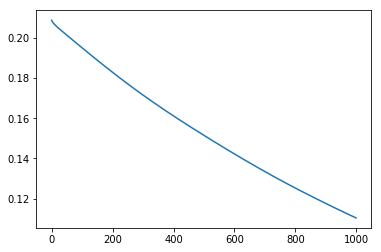The history field of the H object contains all of the model error metrics computed during training. Each metric is its own named list. To pull out just the “loss” data (the loss used to train, which is binary_crossentropy in this case), we specify that name in square brackets–the result is passed as an argument to the plot function, which will render the data as a line. You can see the loss drops smoothely.

After training, the model object contains the learned configuration of weight values. How can you figure out what the model is doing, or what internal representations it has learned, or which items it is getting right, and so on?

One way is to use the predict method of the model object. Predict takes an array of input vectors as an argument, formatted in exactly the same way as the training patterns. For each pattern in the array, it applies the input pattern to the model input units, propagates activity all the way through to the output, and returns the pattern of activation across the output units:

In :
```outacts = model.predict([I,C]) #Generates output activations, stores them in outacts
```

Here I have just asked the model to generate output activations (“predict”) for all of the training patterns. So outacts is now an array containing, for each input pattern, the pattern of activation generated over output units. You can see how many patterns and units it has by calling the .shape method, which returns the shape of the array:

In :
```outacts.shape
```
Out:
`(32, 36)`

The output array contains the output pattern over the 36 output units, for 32 input patterns. We can look at the output pattern generated by using the barplot function in the plt module:

In :
```plt.bar(np.arange(len(outacts[0,])), outacts[0,])
```
Out:
`<BarContainer object of 36 artists>`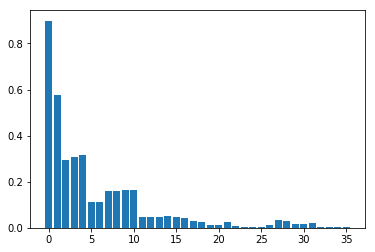Or maybe we want to visualize the difference between the target pattern and the output pattern for a given item:

In :
```plt.bar(np.arange(len(outacts[0,])), T[0,] - outacts[0,])
```
Out:
`<BarContainer object of 36 artists>`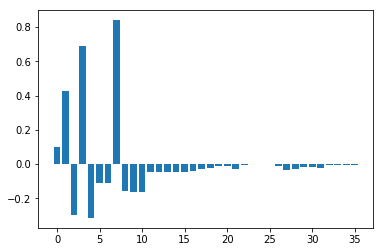We can see that several units are still attracting error (otherwise this difference would be near zero for each unit). We can keep training until the error goes down far enough–just repeat the previous fit command. The model will keep training, beginning with the existing learned weights. Let’s train for 2000 more epochs:

In :
```H = model.fit([I,C], T, epochs = 2000, batch_size = 32)
```
```Epoch 1/2000
32/32 [==============================] - 0s 229us/step - loss: 0.1104 - mean_squared_error: 0.0315 - acc: 0.9575
Epoch 2/2000
32/32 [==============================] - 0s 258us/step - loss: 0.1103 - mean_squared_error: 0.0315 - acc: 0.9575
Epoch 3/2000
32/32 [==============================] - 0s 172us/step - loss: 0.1103 - mean_squared_error: 0.0315 - acc: 0.9575
Epoch 4/2000
32/32 [==============================] - 0s 224us/step - loss: 0.1102 - mean_squared_error: 0.0315 - acc: 0.9575
Epoch 5/2000
32/32 [==============================] - 0s 141us/step - loss: 0.1101 - mean_squared_error: 0.0314 - acc: 0.9575
Epoch 6/2000
32/32 [==============================] - 0s 229us/step - loss: 0.1100 - mean_squared_error: 0.0314 - acc: 0.9575
Epoch 7/2000
32/32 [==============================] - 0s 263us/step - loss: 0.1100 - mean_squared_error: 0.0314 - acc: 0.9575
Epoch 8/2000
32/32 [==============================] - 0s 122us/step - loss: 0.1099 - mean_squared_error: 0.0314 - acc: 0.9575
Epoch 9/2000
32/32 [==============================] - 0s 128us/step - loss: 0.1098 - mean_squared_error: 0.0314 - acc: 0.9575
Epoch 10/2000
32/32 [==============================] - 0s 159us/step - loss: 0.1098 - mean_squared_error: 0.0313 - acc: 0.9575
Epoch 11/2000
32/32 [==============================] - 0s 142us/step - loss: 0.1097 - mean_squared_error: 0.0313 - acc: 0.9575
Epoch 12/2000
32/32 [==============================] - 0s 184us/step - loss: 0.1096 - mean_squared_error: 0.0313 - acc: 0.9575
Epoch 13/2000
32/32 [==============================] - 0s 191us/step - loss: 0.1096 - mean_squared_error: 0.0313 - acc: 0.9575
Epoch 14/2000
32/32 [==============================] - 0s 283us/step - loss: 0.1095 - mean_squared_error: 0.0313 - acc: 0.9575
```

How much has the loss declined?

In :
```plt.plot(H.history['loss'])
```
Out:
`[<matplotlib.lines.Line2D at 0x140910dc438>]`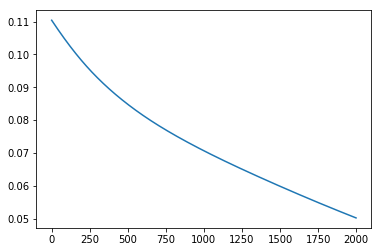Pretty far. How is it doing on pattern 1?

In :
```outacts = model.predict([I,C]) #Generates output activations, stores them in outacts
plt.bar(np.arange(len(outacts[0,])), T[0,] - outacts[0,])
```
Out:
`<BarContainer object of 36 artists>`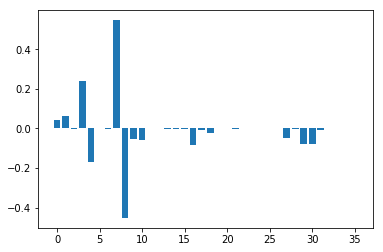Substantially better–all units but 1 are within 0.3 of their target values. (That one unit is the “pine” unit, which is only active for this one input).

But often what we want is to see the patterns generated inside the model hidden layers, to understand how the model is internally representing its inputs. The predict method will take inputs and generate outputs, but how do can we view/analyze/record activations inside the network?

First we need to import some additional tools from Keras, all bundled within the “backend” module. By convention this is abbreviated as K when you import:

In :
```from tensorflow.keras import backend as K #allows us to write keras functions
```

The backend contains a variety of tools for working with components of models. We can use the function procedure in this module to run input through sub-components of our trained model, specifying which model layer is to be used as output by the predict method:

In :
```get_itemreps = K.function([model.layers.input], [model.layers.output])
get_bothreps = K.function([model.layers.input, model.layers.input], [model.layers.output])

ireps = get_itemreps([I]) #The zero at the end pulls the np.array out of the list returned by default
ireps = ireps[0:8,]
breps = get_bothreps([I,C])
#breps
```

K.function calls the function procedure from the Keras backend module and passes arguments indicating which layers in the model object are to be used for receiving input and generating output. The returned object is now a function that can be called on an input array, will apply those inputs to the specified input units, and will return the pattern of activation generated over the specified output layer.

For instance, line I defines a function called get_itemreps that will apply specified input patterns to layer 0 of the model object (the item input layer), then will treat layer 1 (the first hidden layer) as the output. This function can then be applied to an array of input patterns and will return an array of output activations.

Line 4 runs the function on the item training patterns stored in I. NOTE that inputs need to be specified as a list–so you need to enclose the input array (or arrays) in square brackets. Likewise the output is returned as a list with a single element–so you need to pull out the single item in the list by specifying  on the data returned by the function.

In :
```ireps.shape
```
Out:
`(8, 4)`
In :
```from scipy.cluster.hierarchy import dendrogram, linkage

plt.bar(np.arange(len(breps[4,])), breps[4,])
```
Out:
`<BarContainer object of 8 artists>`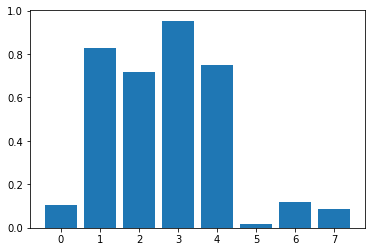In :
```linked = linkage(ireps, 'ward')
plt.figure(figsize=(10, 7))